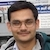# NAEST 2018 Screening Test: Falling SlinkyBy

A slinky (long spring of low spring constant) is suspended vertically by holding one of its end. When released, the slinky gets compressed in a peculiar way. The video (in slow motion) shows the upper end coming down fast whereas the lower end almost remains stationary. After initial compression, the slinky fall down in the compressed form.

Question: The slinky shrinks during fall because

1. It becomes massless during fall.
2. Earth stops pulling it during fall.
3. The slinky pulls the upper coils in the upward direction but it pulls the lower coil in the downward direction.
4. The slinky pulls the upper coils in the downward direction and the lower coils in the upward direction.

Solution: Let us see the forces acting on the upper coil (U) and the lowe coil (L) of the slinky. The forces on the upper coil when it is suspended from support (hand) are

1. downward spring force ($F$)
2. downward weight ($mg$)
3. upward reaction from the support ($R$)
Initially, the net force on the upper coil is zero i.e., $R=mg+F$. When released, the upper coil U loose contact with the support making $R=0$. The spring force $F$ continues to pull the upper coil U downwards. The net force on this coil is $mg+F_U$ (downward). The downward acceleration of the upper coil is \begin{align} a_U=g+F/m. \end{align}Similarly, the forces on the lower coil L are

1. upward spring force ($F$)
2. downward weight ($mg$)
Initially, the coil is at rest i.e., $F=mg$. When released, the spring force $F$ continues to pull the lower coil L upwards. The downward acceleration of the lower coil is \begin{align} a_L=g-F/m. \end{align}

Note that $a_U>a_L$. Thus, the upper coil moves down fast in comparison to the lower coil and the slinky gets compressed. The magnitude of the spring force $F$ decreases continuously from its initial value to almost zero (due to spring compression).

After initial compression, the spring force becomes zero ($a_U=a_L=g$). The slinky falls freely in the compressed form with an acceleration $g$.